# Collision point of two non-rotating polygons

### March, 1995

A couple of threads recently on Compuserve have dealt with how to determine when two non-rotating polygon objects collide. Below is a short discussion of one algorithm to determine when and where the collision happens. The algorithm will work for both convex and concave figures with any number of line segments. The algorithm works entirely by intersecting lines, and thus does not rely on time-consuming iterative methods. The algorithm works even for objects that pass completely through each other in the course of one frame.

There are a few special cases we need to handle. First, the math goes to infinity when we compare two line segments which are parallel or nearly-parallel. Also, the math gets funky if one of the parallel lines doesn't move this frame.Similarly, if either of the lines are vertical then the slope goes to infinity.

I don't get into rotating polygons, transferring momentum between the objects (see my ricochet discussion for ball collisions. Much of that info will apply to this as long as the objects are nearly circular,) accelerations during the frame, nor multiple collisions within one frame of action.

The trick to making this simple and quick is to recognize that the "point of intersection" between the two line segments changes over the course of the frame. The path of the point of intersection describes a line. Intersect that line with the line described by the path of one of the endpoints and you have a possible collision point. This is fairly difficult to explain in words, but the figures included in this page will help you visualize what's happening.

Prior to Figure 1 you'll want to perform some bounding box tests to cull out most of the line segments on the screen. First, you'll want to test each object against each other object. Be sure to include the full extents of the objects motion for the current frame. Next, for any object pairs which pass that test you'll want to test each line segment in one object with each line segment in the other object. In most situations you'll probably be down to a very few lines to compare.

Figure 1 shows two objects and their velocity vectors.Figure 2 highlights one line segment on each object.In Figure 3, we've extended the line segments indefinitely in each direction. This gives us our initial point of intersection.

For those who are years from their last algebra class, here are the equations for intersecting two lines:

```x=(m2*x2-m1*x1-y2+y1)/(m2-m1); // m's are slopes, x's & y's are pts on lines```
`y=m1*(x-x1)+y1; // point/slope form`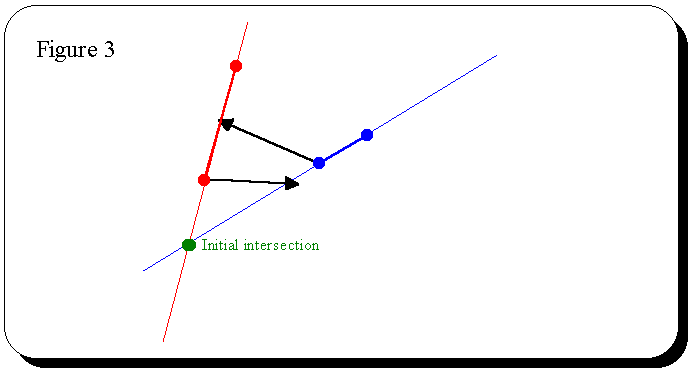In Figure 4, we've moved the line segments to their position at the end of the frame and again extended the lines. This time we get the final point of intersection. Over the course of the frame, the path of this point of intersection will describe a line from the initial to the final point.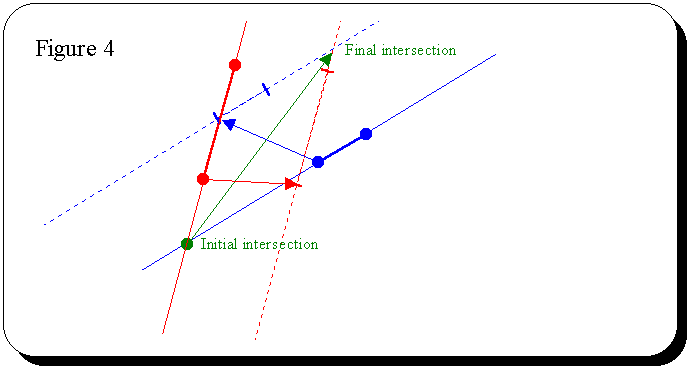In Figure 5, we've shown the path of the endpoints and where they intersect with path of intersection. We end up with four possible points of collision. At this point, you probably want to sort the four points by distance to the initial initial point of intersection. The closest happens earliest in the frame (neglecting acceleration.)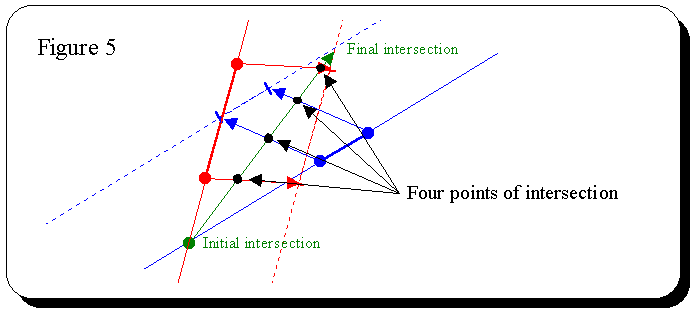In Figure 6, we've determined that Point 1 lies outside of the blue line segment. The easiest way to determine this is to calculate the distance from Point 1 to each of the endpoints of the blue line segment. If either distance is greater than the length of the blue line segment then the point lies outside the line segment.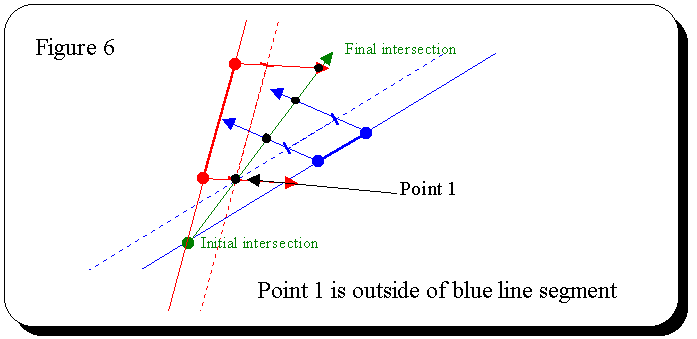In Figure 7, we've determined that Point 2 does lie within the red line segment. This is our point of impact. The distance along the path of intersection is proportional to the time within the frame when the collision occurred. IOW, in Figure 7, Point 2 appears to lie just about halfway from the Initial point to the Final point. Therefore, the collision happened halfway through the frame (again, neglecting acceleration.)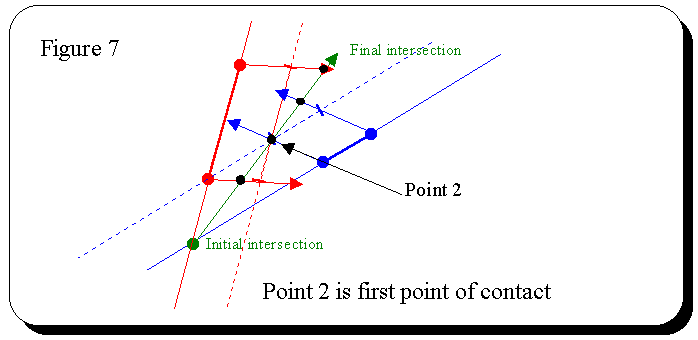That's all there is to it. The figures make it pretty straightforward.

## Special cases:

You may remember that I mentioned special cases. If the line segments in question are parallel then you won't have a path of intersection to play with. Instead, the lines containing the line segments will overlap at only one instant during the frame.

Another, problem arises if the lines are nearly parallel. In that case, the Initial and Final points of intersection may be so far away that you risk overflowing your variables. I suggest that you treat nearly parallel lines as parallel and perform the following special case algorithm.

The trick for parallel lines is to recognize that the fact that they're parallel in effect removes one dimension from the problem. We've gone from a two-dimensional system with intersection lines to a one-dimensional system where two lines are closing on each other.

Actually, they may not be headed directly at each other, as shown in Figure 8.But, since the lines are known to be parallel, then the velocity vector perpendicular to the line's slope is by definition the closing speed relative to the other line segment. These are the dotted lines in Figure 9. Once we know these closing speeds, the problem becomes a simple one-dimensional rate-distance problem.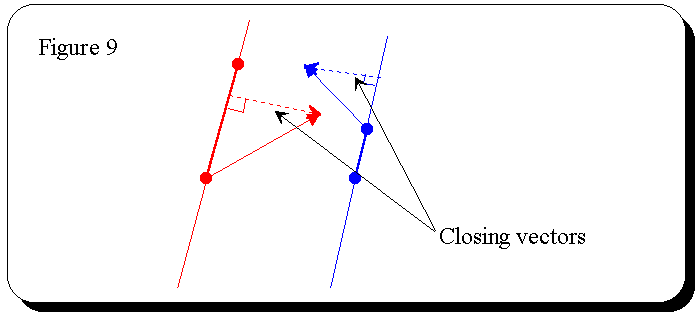`rate1 * t + rate2 * t = distance `
`t = distance / (rate1 + rate2) `

Once you've calculated t you need to test the line segments to verify that they have in fact overlapped. Take one endpoint from the red line segment and calculate the distance to both endpoints of the blue line segment. If the distance to both is less than the length of the blue line segment then the red endpoint falls inside the blue line segment and you have a successful collision. Otherwise, perform the same test with all the other endpoints. If any of the endpoints falls on the other line segment, then you have a successful collision.

If one of these parallel lines isn't moving then you end up with a divide-by-zero situation. Simply perform the perpendicular calculation only for the moving line. Then the distance formula will work correctly.

Finally, vertical lines. If both are vertical then it's simply a parallel line situation like I just described.

If only one of the lines is vertical, then you need to use a different intersection equation. Simply compute the y-intercept.

`y = mx + b`
`b = y - mx `
`Where l1 is the vertical line`
`y is a point on l2`
```x is the distance from that point to l1 (subtract the x of that point from the x of the vertical line)```
`m is the slope of l2`

Remember, even though one line is vertical, it's still moving. So you need to move both lines for beginning and ending intersection points.

## Optimizations:

At the beginning of this document I mentioned using bounding boxes to cut down the number of lines to test. Bounding box tests are cheap, and even with 50+ objects on the screen the time spent comparing bounding boxes will probably be completely swamped by simply drawing things on the screen. You'll only need something more sophisticated when you get a lot more objects on the screen.

Similarly, once you get two entire objects which satisfy the bounding box test, you'll likely only end up with a small number of line segments which actually need to be tested with each other.

A couple of quick optimization ideas in the calculations. These will happen so rarely that we probably wouldn't even notice the extra work. But they're so obvious I just had to mention them.

In Figure 5, where we compare the distance to each of the possible collision points. In this instance, we don't need to know the actual distance. We simply need the ranking from lowest to highest. Therefore, we don't need to take the square root of each of those calculations. When it comes time to determine the time within the frame then we will need to take the square root.

Similarly, we don't need to take the square root when determining if the possible point of impact is on the line segment. Compare against the square of the length of the line segment.

Well, I think that's enough to get you started. I'd like to throw together some example code, but I'm completely swamped at work, so I'll leave that as an exercise. If anyone posts some code that's based on these ideas, please let me know and I'll include a pointer in this document.

## References:

Be sure to check out IMPACT21.ZIP by John Henckel. It's available in GAMDEV on Compuserve and shows up on internet searches. It's a nifty polygon collision example, including source code. He handles rotating polygons, and momentum transfer.

Thanks to Kevin J Hoffman for spurring this line of thought and brainstorming with me. It was an interesting problem.

Drake Christensen mighty@mightydrake.com.

##### Please email comments, typos, errors, dead links, and any suggestions to webmaster@mightydrake.com. (Privacy statement) Copyright © 1997-2007 Mighty Drake, Inc. All rights reserved. Last modified: January 25, 2003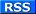Best viewed with: Hosted by: Composed with: In association  with: Fight Spam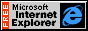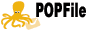Opera or Mozilla or Explorer or Netscape Site 5 FrontPage Amazon.com Spamcop.net &  Popfile & Greylisting